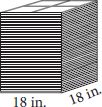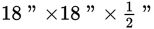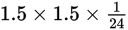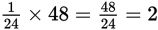# SAT Math Multiple Choice Question 981: Answer and Explanation

### Test Information

Question: 981

1.A flooring company stores its marble tiles in vertical stacks as shown above. Eachtile measures. How many cubic feet of tile are there in one stack of 48 of these tiles?

• A. 4.5
• B. 54
• C. 162
• D. 648

Getting to the Answer: It is easier to convert inches to feet than cubic inches to cubic feet, so start by converting each of the dimensions of the tile to feet. There are 12 inches in 1 foot, so the dimensions of a single piece of tile are (18 ÷ 12) × (18 ÷ 12) × (0.5 ÷ 12) or, when simplified,. The stack of tiles takes on the shape of a right rectangular prism, so you can find the volume using the formula V = lwh. You already know the length (1.5 ft) and the width (1.5 ft). To find the height, multiply the height of one tile by the number of tiles in the stack:feet. This means the number of cubic feet in the stack of tiles is 1.5 × 1.5 × 2 = 4.5, making (A) correct.